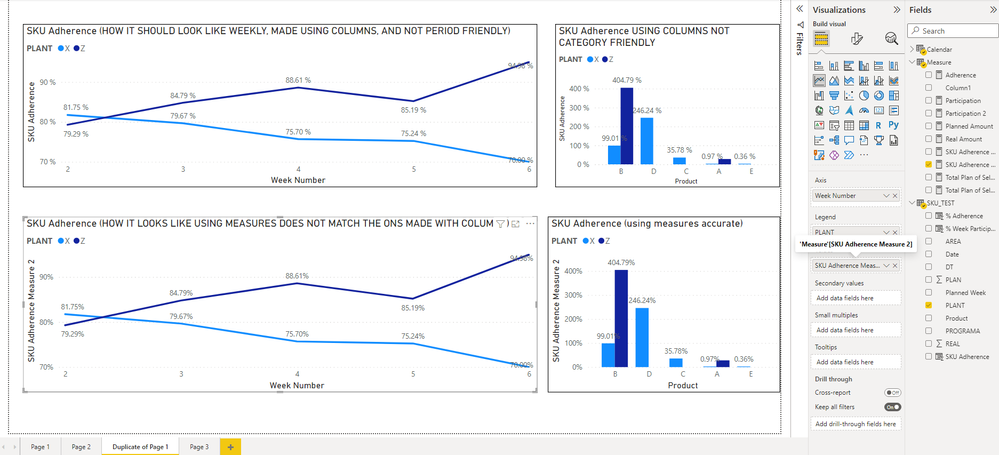cancel
Showing results for
Did you mean:Helper I

## Combined iteration of two measures ( multiply row by row between two measures)

Hello,

Currently migrating a KPI (adherence by SKU) from Excel to PBI and having difficulties, here is the definition:Where:Made a replica using columns but is not what im looking for because it is not scalable, or relative to other categories and only works as intended using the participation baseline ( currently a week):Made a version with measures and it not working properly even on weeks:
Here is the comparison:Here are my Measures:

``````Planned Amount =
If(
CALCULATE(SUMX(SKU_TEST,SKU_TEST[PLAN]),DATESYTD(SKU_TEST[Date]))=0,
BLANK(),
CALCULATE(SUMx(VALUES(SKU_TEST[PLAN]),Calculate(SUM(SKU_TEST[PLAN]),DATESYTD(SKU_TEST[Date])))))

//////////////////////////////////////////////////////////////////////////////////////////

Real Amount =
If(
CALCULATE(SUMX(SKU_TEST,SKU_TEST[PLAN]),DATESYTD(SKU_TEST[Date]))=0,
BLANK(),
CALCULATE(SUMx(VALUES(SKU_TEST[REAL]),Calculate(SUM(SKU_TEST[REAL]),DATESYTD(SKU_TEST[Date])))))

///////////////////////////////////////////////////////////////////////////////

If(
[Planned Amount]=0,
BLANK(),
IF(
DIVIDE([Real Amount],[Planned Amount])>1,
1,
DIVIDE([Real Amount],[Planned Amount])))

////////////////////////////////////////////////////////////////////////

Total Plan of Selected Period =
If(
[Planned Amount]=0,
BLANK(),
CALCULATE([Planned Amount] ,
ALLEXCEPT(SKU_TEST,
SKU_TEST[Date],SKU_TEST[PROGRAMA],SKU_TEST[DT],SKU_TEST[Product],SKU_TEST[AREA], SKU_TEST[PLANT], 'Calendar'[Date],'Calendar'[Week Number],'Calendar'[Month],'Calendar'[Year])
))

//////////////////////////////////////////////////////////////////////////////////

Participation =
If(
[Planned Amount]=0,
BLANK(),
DIVIDE([Planned Amount],[Total Plan of Selected Period]))

///////////////////////////////////////////////////////////////////////////////////////

Basically Im looking to get the result from the columns in the weeks graphs but with the flexibility of Measures.

Attaching data samples and Test File https://we.tl/t-U21qCp613h

Best Regards

RT

1 ACCEPTED SOLUTIONCommunity Support

Hi @RTERCERO ,

Change your corresponding measures like below：

``````Total Plan of Selected Period 2 =
CALCULATE (
SUM ( SKU_TEST[PLAN] ),
ALLEXCEPT ( SKU_TEST, SKU_TEST[PLANT], SKU_TEST[Planned Week] )
)
``````
``````Participation 2 =
IF (
[Planned Amount] = 0,
BLANK (),
DIVIDE ( [Planned Amount], [Total Plan of Selected Period 2] )
)
``````
``````SKU Adherence Measure 2 =
VAR t_ =
RETURN
SUMX ( t_, [a_] * [b_] )
``````Best Regards,

Icey

If this post helps, then please consider Accept it as the solution to help the other members find it more quickly.

3 REPLIES 3Community Support

Hi @RTERCERO ,

Change your corresponding measures like below：

``````Total Plan of Selected Period 2 =
CALCULATE (
SUM ( SKU_TEST[PLAN] ),
ALLEXCEPT ( SKU_TEST, SKU_TEST[PLANT], SKU_TEST[Planned Week] )
)
``````
``````Participation 2 =
IF (
[Planned Amount] = 0,
BLANK (),
DIVIDE ( [Planned Amount], [Total Plan of Selected Period 2] )
)
``````
``````SKU Adherence Measure 2 =
VAR t_ =
RETURN
SUMX ( t_, [a_] * [b_] )
``````Best Regards,

Icey

If this post helps, then please consider Accept it as the solution to help the other members find it more quickly.Helper I

Hello @Icey ,

Thanks for the help, this solution works to replicate whats done using columns however, It takes a lot more time to calculate and still does not the flexibility when changing between Days/ Weeks/ Months, Works on weeks only.

Q1 Is there another option to make it Faster?

Q2 can We make it flexible between Time periods?

I was considering maybe in "Total Plan of Selected Period 2" Is where I have the Oportunity.

What do you think?

RTHelper I

Adding an "ALLSELECTED( SKU_TEST )", did the trick here: "Total Plan of Selected Period 2"